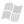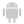Home |Sign in | EnglishHelpWLanguageWLanguage functionsControls, pages and windowsSpreadsheet functionsObject syntaxAddFormulaPresentationExampleRelated examplesSee alsoAddDataAddFormulaAddImageAddNameAddWorksheetAllowProcedureBorderSelectionCloseControlFormulaDeleteAllDeleteColumnDeleteLineDeleteWorksheetEndModificationFreezeGetFormulaGetMergeGetSelectionInsertColumnInsertRowInsertWorksheetLoadPositionRecalculateAllReplaceRestorePositionAndSelectionSaveSavePositionAndSelectionSeekSelectMinusSelectPlusSelectWorksheetSortSelectionStartModificationStyleSelectionTypeAndMaskSelectionSpreadsheet functions (object syntax)
WINDEVWEBDEVWINDEV MobileOthersIn french: <Tableur>.AjouteFormule
Adds a calculation formula into a Spreadsheet control found in a window.
Example
//  Initialize the 1st column with numbers from 1 to 10
FOR I = 1 TO 10
END

Syntax

Adding a formula into a cell identified by its coordinates

Name of Spreadsheet control to use.
<Column>: Integer
Column number of the cell containing the formula.
<Row>: Integer
Row number of the cell containing the formula.
<Formula>: Character string (with quotes)
Formula to add. The first "=" character must not be included in the formula. For example: "A1+B2-A2"
In case of syntax error or invalid identifier, "###" is displayed in the cell and a non-fatal error is displayed.

Adding a formula into a cell identified by its name

Name of Spreadsheet control to use.
<Cell name>: Character string (with quotes)
Name of cell to use. For example: "A4".
<Formula>: Character string (with quotes)
Formula to add. The first "=" character must not be included in the formula. For example: "A1+B2-A2"
In case of syntax error or invalid identifier, "###" is displayed in the cell and a non-fatal error is displayed.
Remarks
• If the cell (Column, Row) does not exist, the cell is created.
• If the cell (Column, Row) already exists, the content is deleted. It is replaced by the <Formula> formula.
• This function can only be used on a Spreadsheet control found in a window.
•Versions 22 and later
The cells can be renamed (<Spreadsheet>.AddName). You have the ability to use the name of a cell in the formula.New in version 22
The cells can be renamed (<Spreadsheet>.AddName). You have the ability to use the name of a cell in the formula.
The cells can be renamed (<Spreadsheet>.AddName). You have the ability to use the name of a cell in the formula.
Related Examples:Unit examples (WINDEV): The Spreadsheet control [ + ] Using the Spreadsheet control.This example explains how to:- load an xlsx file in a spreadsheet control,- save the spreadsheet in a file,- fill the control with data coming from the database,- insert rows, columns,- access the cells and handle them (modify their value, their style, ...),- enter formulas,- ...
Component : wd240obj.dll
Minimum required version
• Version 23